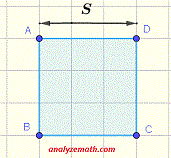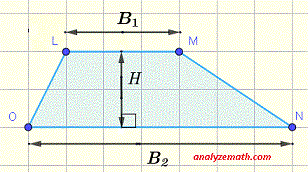# Calculate Areas of Squares, Rectangles, Triangles, Parallelograms and Trapezoids - Grade 6

Grade 6 examples and questions to calculate areas of rectangles, squares, triangles, parallelograms and trapezoids with detailed solutions and explanations are presented.

Formulas to Calculate Areas

The formulas to calculate the areas of rectangles, squares, triangles, parallelograms and trapezoids are presented.. Rectangle: Area = L × W. Square: Area = S × S = S 2. Triangle: Area = (1 / 2) × H × B. Parallelogram: Area = L × H. Trapezoid: Area = (1 / 2) × H × (B1 + B2)

More High School Math (Grades 10, 11 and 12) - Free Questions and Problems With Answers
More Middle School Math (Grades 6, 7, 8, 9) - Free Questions and Problems With Answers
More Primary Math (Grades 4 and 5) with Free Questions and Problems With Answers